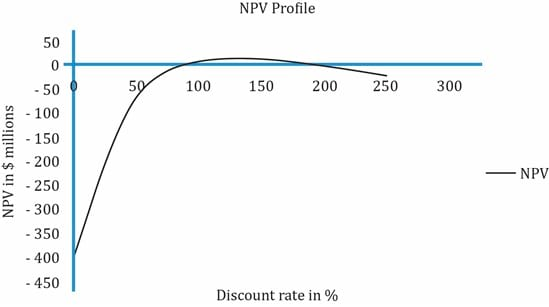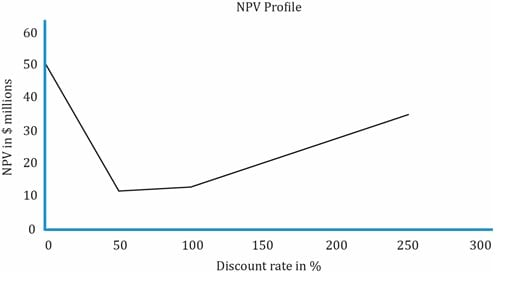IFT Notes for Level I CFA® Program
IFT Notes for Level I CFA® Program

# Part 4

### 4.8. Ranking Conflicts between NPV and IRR

For single and independent projects with conventional cash flows, there is no conflict between NPV and IRR decision rules. However, for mutually exclusive projects the two criteria may give conflicting results. The reason for conflict is due to differences in cash flow patterns and differences in project scale.

Example (Ranking conflict due to differing cash flow patterns)

The cash flow associated with Project X and Project Y is shown below:

 Year 0 1 2 3 4 Project X -400 160 160 160 160 Project Y -400 0 0 0 800
1. Which project do you select according to the NPV rule using a rate of 10%?
2. Which project do you select according to the IRR rule?
3. Show the NPV profile for both projects.

Solution:

Let us first calculate the NPV and IRR for the two projects.

 NPV (in \$ millions) IRR (in %) Project X 107.17 21.86 Project Y 146.4 18.92
1. Based on the NPV rule, the project with the highest NPV, project Y is selected.
2. Based on the IRR rule, the project with the highest IRR, project X is selected.
3. We saw the NPV profile for both projects in the previous example (crossover point). Whenever NPV and IRR rank two mutually exclusive projects differently, you must always choose the one with the higher NPV – in this case, project Y.

Reasons for going with NPV instead of IRR:

1. IRR incorrectly assumes that intermediate cash flows can be reinvested at the IRR rate. Just because project X gives a return of 21.86%, it does not mean the intermediate cash flows can be reinvested at that rate.
2. NPV uses a realistic discount rate assumption of 10%. It is the opportunity cost of funds. You can easily find other projects to invest that will give a return of 10%. Hence it is safe to assume that the intermediate cash flows can be reinvested at this rate. The NPV assumes that cash flows are reinvested at the required rate of return.

Besides differences in cash flow patterns, there can be ranking conflicts due to differences in project size as well. Consider two projects one with an initial outlay of \$1 million and another project with an initial outlay of \$1 billion. It is possible that the smaller project has a higher IRR, but the increase in firm value (NPV) is small as compared to the increase in firm value (NPV) of the larger project.

### 4.9. The Multiple IRR Problem and No IRR Problem

If a project has unconventional cash flows, it can have multiple IRRs, i.e., there are more than one discount rates that will produce an NPV equal to zero. The NPV profile of a project with multiple IRRs intersects the x-axis at more than one point.Some projects do not have an IRR, i.e. there is no discount rate that results in a zero NPV. No IRR projects may have positive NPVs and can be good investments. However, because of unconventional cash flows, mathematically no IRR exists. The NPV profile of a project with no IRR does not intersect the x-axis.Comparison between NPV and IRR

 NPV IRR Advantages Advantages Direct measure of expected increase in value of the firm. Shows the return on each dollar invested. Theoretically the best method. Allows us to compare return with the required rate. Disadvantages Disadvantages Does not consider project size. Incorrectly assumes that cash flows are reinvested at IRR rate. The correct assumption is that intermediate cash flows are reinvested at the required rate. Might conflict with NPV analysis. Possibility of multiple IRRs or no IRR for a project.

### 4.10. Popularity and Usage of the Capital Budgeting Methods

Analysts and corporate managers should understand the logic and practicalities of different capital budgeting methods.

From financial textbooks perspective, NPV and IRR are superior techniques. However, companies also prefer other capital budgeting techniques, e.g., payback period.

Relationship between NPV and Stock Price

• The value of a company can be measured as the existing value plus the present value of its future investments. NPV is a direct measure of the expected change in the firm’s value from undertaking a capital project.
• A positive NPV project should cause a proportionate increase in a company’s stock price. But, if the project’s profitability is less than expectations, then the stock price may be negatively impacted.

Example

A company is undertaking a project with an NPV of \$500 million. The company currently has 100 million shares outstanding and each share has a price of \$50. What is the likely impact of the project on the stock price?

Solution:

NPV of the project = \$500 million. The overall value of company should increase by \$500 million because of the project. Since there are 100 million shares outstanding, each share should go up by 500/100 = \$5. The share price should increase from \$50 to \$55.